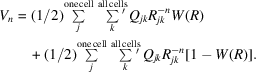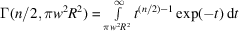International
Tables for
Crystallography
Volume B
Reciprocal space
Edited by U. Shmueli

International Tables for Crystallography (2010). Vol. B, ch. 3.4, pp. 449-450   | 1 | 2 |

Section 3.4.3. Preliminary description of the method

D. E. Williamsa

aDepartment of Chemistry, University of Louisville, Louisville, Kentucky 40292, USA

3.4.3. Preliminary description of the method

| top | pdf |

Ewald (1921) developed a method which modified the mathematical representation of the Coulombic lattice sum to improve the rate of convergence. This method was based on partially transforming the lattice sum into reciprocal space. Bertaut (1952) presented another method for derivation of the Ewald result which used the concept of the crystallographic structure factor. His formula extended the Ewald treatment to a composite lattice with more than one atom per lattice point. Nijboer & DeWette (1957) developed a general Fourier transform method for the evaluation ofsums in simple lattices. Williams (1971) extended this treatment to a composite lattice and gave general formulae for thesums for any crystal. A review article, on which this chapter is based, appeared later (Williams, 1989a,b).

Consider a function, W(R), which is unity atand smoothly declines to zero as R approaches infinity. If each term of the lattice sum is multiplied by W(R), the rate of convergence is increased. However, the rate of convergence of the remainder of the original sum, which contains the difference terms, is not increased.In the accelerated-convergence method the difference terms are expressed as an integral of the product of two functions. According to Parseval's theorem (described below) this integral is equal to an integral of the product of the two Fourier transforms of the functions. Finally, the integral over the Fourier transforms of the functions is converted to a sum in reciprocal (or Fourier-transform) space. The choice of the convergence function W(R) is not unique; an obvious requirement is that the relevant Fourier transforms must exist and have correct limiting behaviour. Nijboer and DeWette suggested using the incomplete gamma function for W(R). More recently, Fortuin (1977) showed that this choice of convergence function leads to optimal convergence of the sums in both direct and reciprocal space:whereandare the gamma function and the incomplete gamma function, respectively:andThe complement of the incomplete gamma function isReferences

Bertaut, E. F. (1952). L'énergie électrostatique de réseaux ioniques. J. Phys. (Paris), 13, 499–505.
Ewald, P. P. (1921). Die Berechnung optischer und elektrostatischer Gitterpotentiale. Ann. Phys. (Leipzig), 64, 253–287.
Fortuin, C. M. (1977). Note on the calculation of electrostatic lattice potentials. Physica (Utrecht), 86A, 574–586.
Nijboer, B. R. A. & DeWette, F. W. (1957). On the calculation of lattice sums. Physica (Utrecht), 23, 309–321.
Williams, D. E. (1971). Accelerated convergence of crystal lattice potential sums. Acta Cryst. A27, 452–455.
Williams, D. E. (1989a). Accelerated convergence treatment of R−n lattice sums. Crystallogr. Rev. 2, 3–23.
Williams, D. E. (1989b). Accelerated convergence treatment of R−n lattice sums. Corrections. Crystallogr. Rev. 2, 163–166.